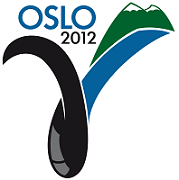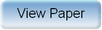Ninth International Geostatistics Congress, Oslo, Norway
June 11 – 15, 2012Session: Theory 3 Abstract No.: O-069 Title: A Review of Probability Aggregation Methods in Earth Sciences Author(s): A Comunian, University of New South Wales/NCGRT (AU)D. Allard, INRA, Avignon (FR)P. Renard, University of Neuchatel (CH) Abstract: Combining different sources of information is a frequent problem in Earth sciences. It occurs for example when modeling a reservoir using direct geological observations, geophysics, or remote sensing. From each source of information one can model the probability of occurrence of a certain event (e.g. the presence of a certain lithofacies at a certain location). The combination of the different sources of information into a single model is then the problem of aggregating different probability distributions. This problem is obviously ill posed since a part of the information is usually missing: only some conditional probabilities are available but not the complete joint probability model. In this framework, we provide an overview of most of the techniques proposed in the literature to aggregate probability distributions. This includes the linear opinion pool, the Beta-transformed linear pool, Bordley's formula, the Tau and the Nu model, or a Maximum Entropy principle. The methods are classified according to their main properties: convexity, response to the impossible or certain event, and external Bayesianity. This classification will help the user to select a method according to its desired properties. The methods are then compared in the perspective of Earth sciences applications on several examples using the notions of reliability and sharpness. The main results are the following. While many methods are equivalent (e.g. Bordley's formula and Tau model) when restricted to the binary case, there is an important difference when considering more than two possible outcomes. When optimized weighting factors can be computed, the best results are obtained with the Beta-transformed linear pool and Bordley's formula. When optimized weights cannot be computed, and when the hypothesis of conditional independence among the various sources of information is justified, good results are obtained with the Maximum Entropy principle and the Bordley's formula with unitary weights.Produced by X-CD Technologies Inc.